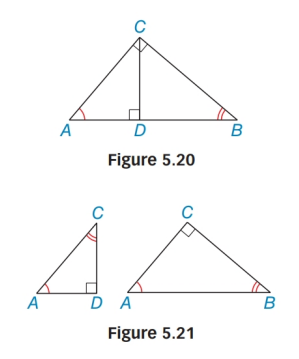Chapter 5.4, Problem 44EElementary Geometry For College St...

7th Edition
Alexander + 2 others
ISBN: 9781337614085

Solutions

Chapter
SectionElementary Geometry For College St...

7th Edition
Alexander + 2 others
ISBN: 9781337614085
Textbook Problem

Use Figures 5.20 and 5.21 to prove Lemma 5.4.3.Lemma 5.4.3The length of each leg of a right triangle is the geometric mean of the length of the hypotenuse and the length of the segment of the hypotenuse adjacent to that leg.

To determine

To prove :

The lemma 5.4.3 “The length of each leg of a right triangle is the geometric mean of the length of the hypotenuse and the length of the segment of the hypotenuse adjacent to that leg”.

Explanation

Given figures 5.19 and 5.20

From the figure ΔABC, with right ACB; CD¯AB¯

Now we have to prove ABAC=ACAD.

By forming a table,

 1 ΔABC with right ∠ACBCD¯⊥AB¯ Given 2 CD¯ is an altitude to AB¯ AN altitude is a segment from a vertex and perpendicular the opposite side

Still sussing out bartleby?

Check out a sample textbook solution.

See a sample solution

The Solution to Your Study Problems

Bartleby provides explanations to thousands of textbook problems written by our experts, many with advanced degrees!

Get Started

Find the domain of each function. 19. (a) f(x)=1ex21e1x2 (b) f(x)=1+xecosx

Single Variable Calculus: Early Transcendentals, Volume I

What is 834 of \$12,000?

Elementary Technical Mathematics

In Problems 4-6, use the function . 5. Find the points of inflection of this function.

Mathematical Applications for the Management, Life, and Social Sciences

Use the Taylor polynomial of degree 2 for centered at 1 to estimate . 1.250 1.255 1.265 1.270

Study Guide for Stewart's Single Variable Calculus: Early Transcendentals, 8th